The Learning Point‎ > ‎Chemistry‎ > ‎

### Problems related to Hybridization and Isomers

 PREVIOUS YEAR JEE PROBLEMS ON NOMENCLATURE, BONDING, HYBRDISATION & ISOMERISMAmongst the given options, the compound(s) in which all the atoms are in one plane in all the possible conformations(if any), is/are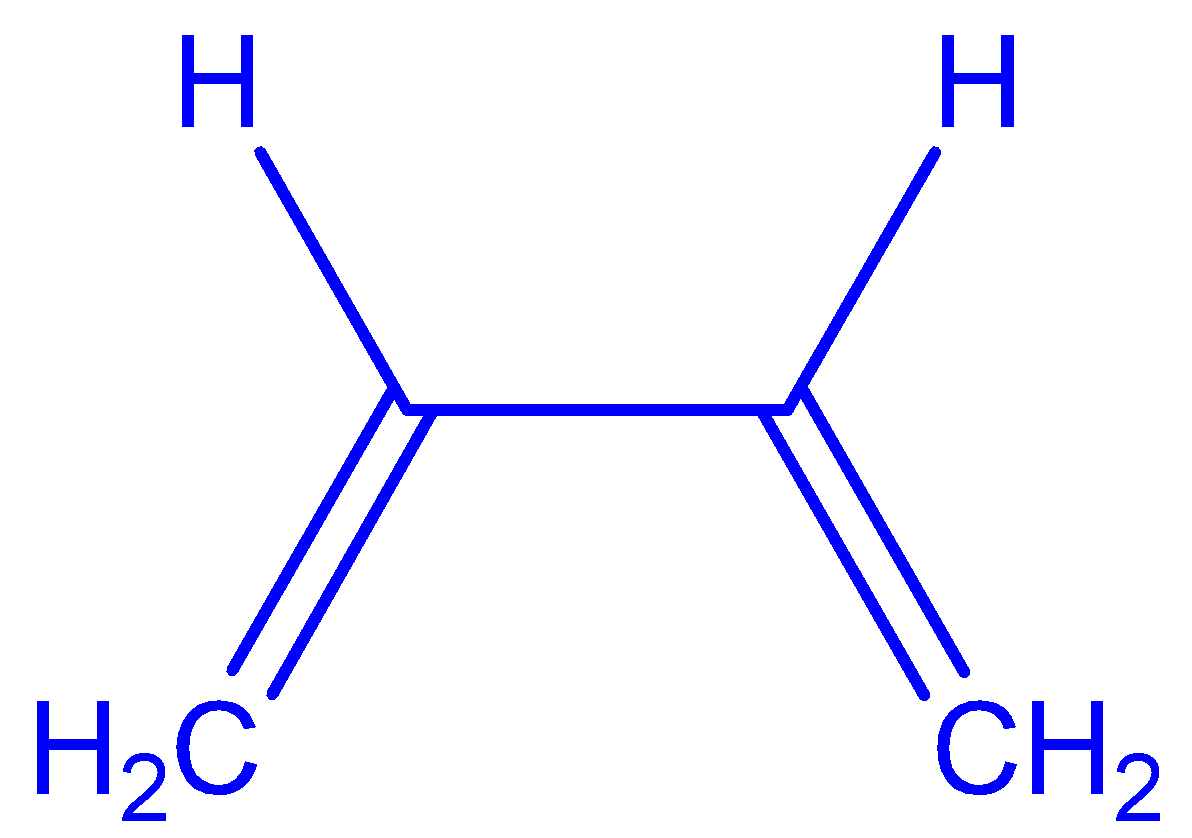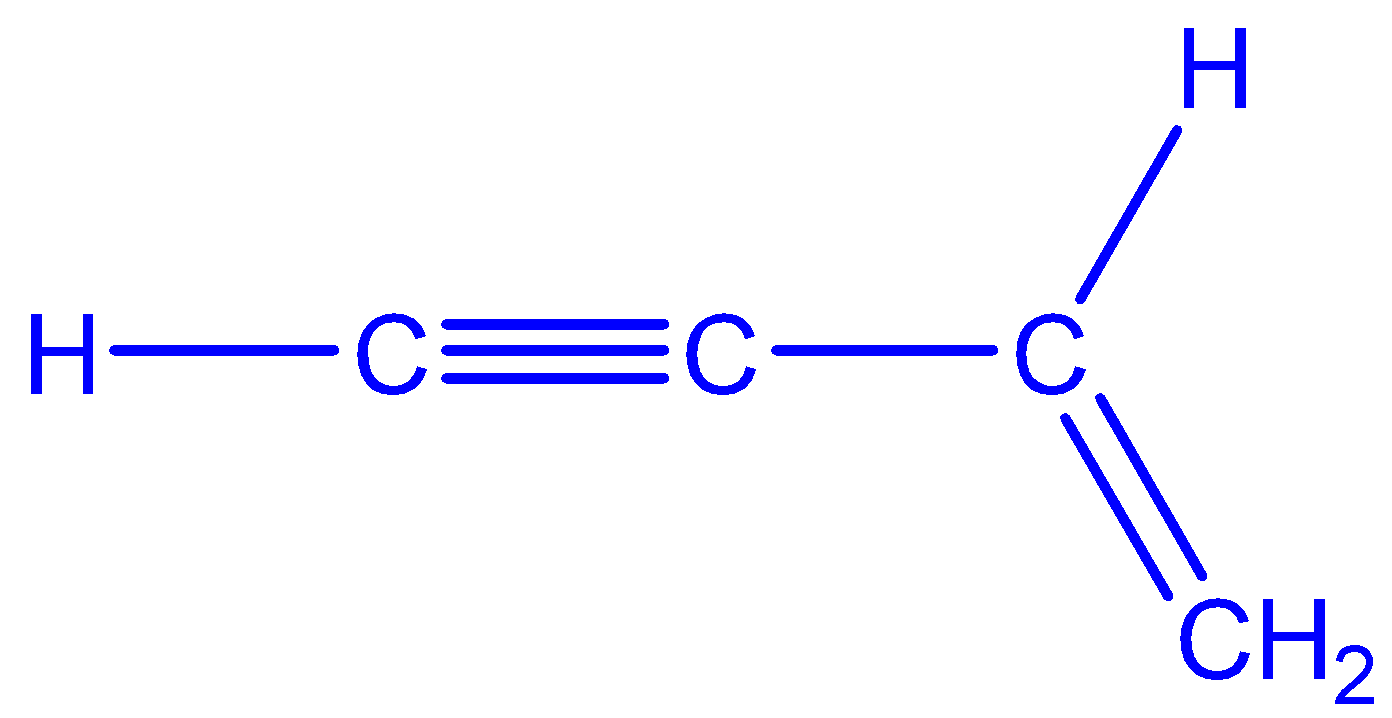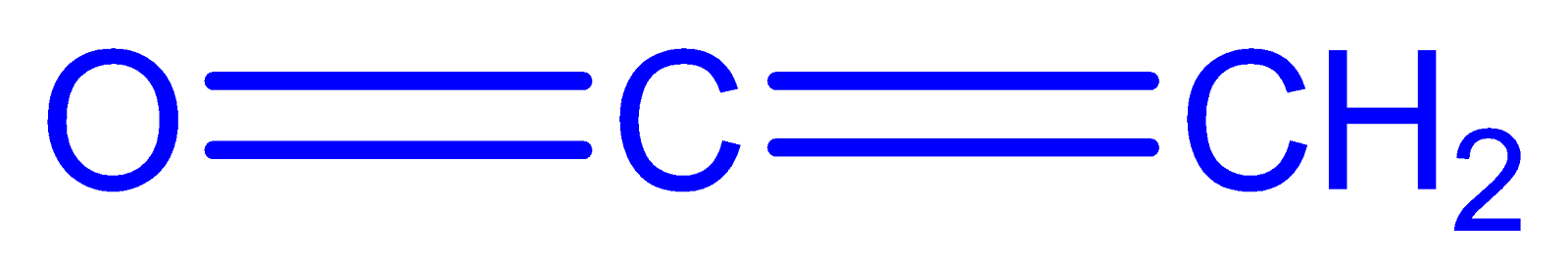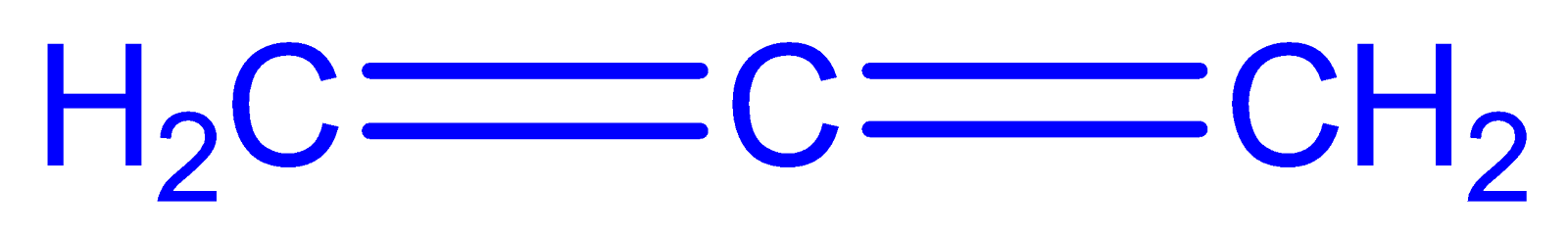Ans: (b) & (c)In (a) free rotation is possible around C2-C3 while (d) is an allene in which the two double bonds are perpendicular w.r.t. each other. As we know, sp2 hybridised structure is planar.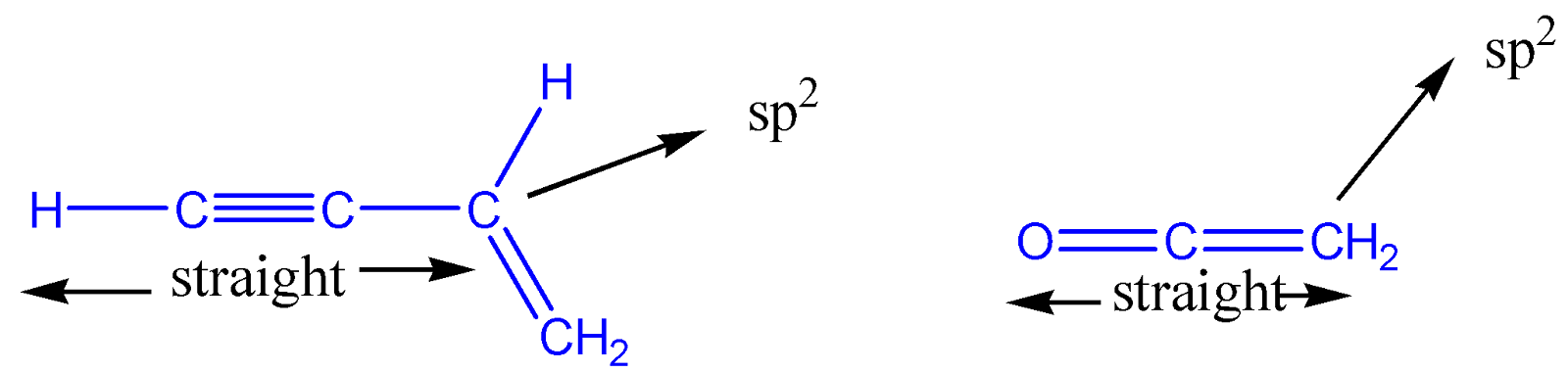The max. no. of isomers (structural + stereo) that are possible on the monochlorination of the following compound is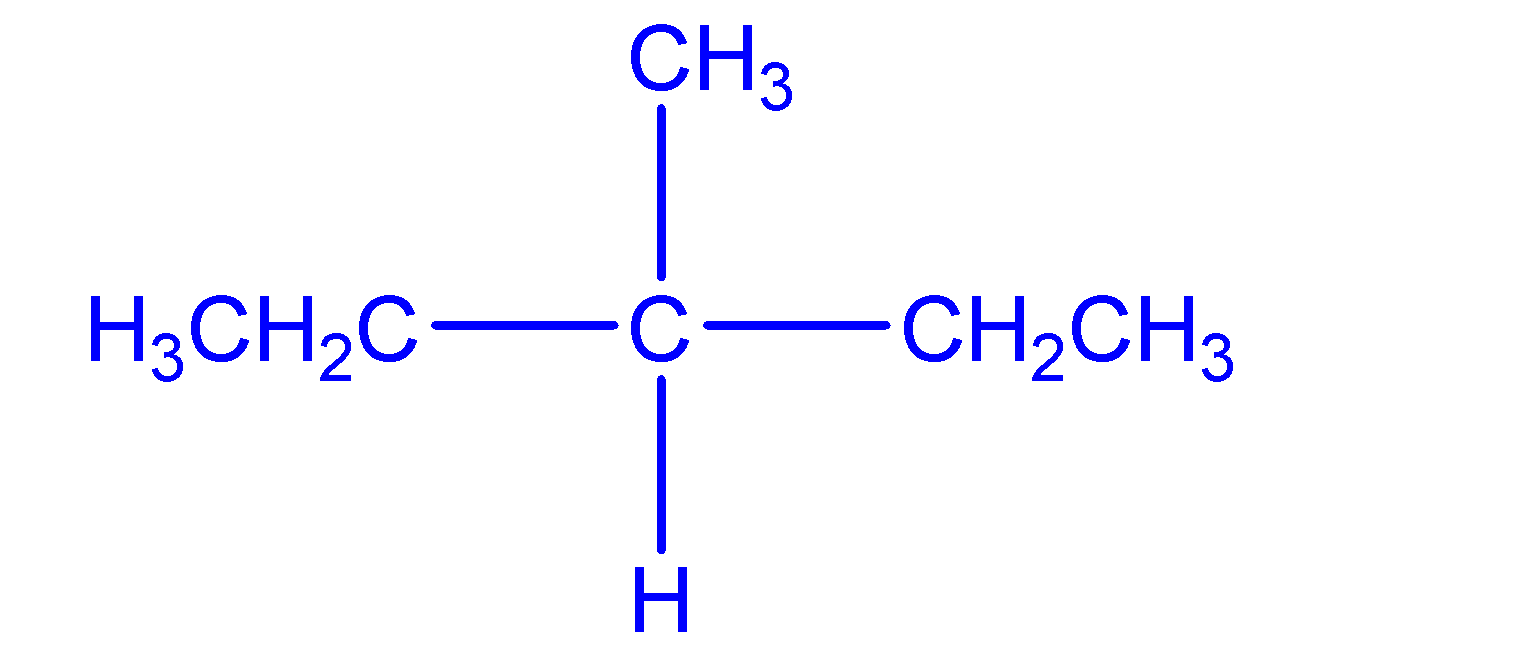Ans: There are 4 different kinds of hydrogen present in the compound. So, we have got 4 structural isomers on substitution of these 4 hydrogens and no. of structural isomers will be equal to 4.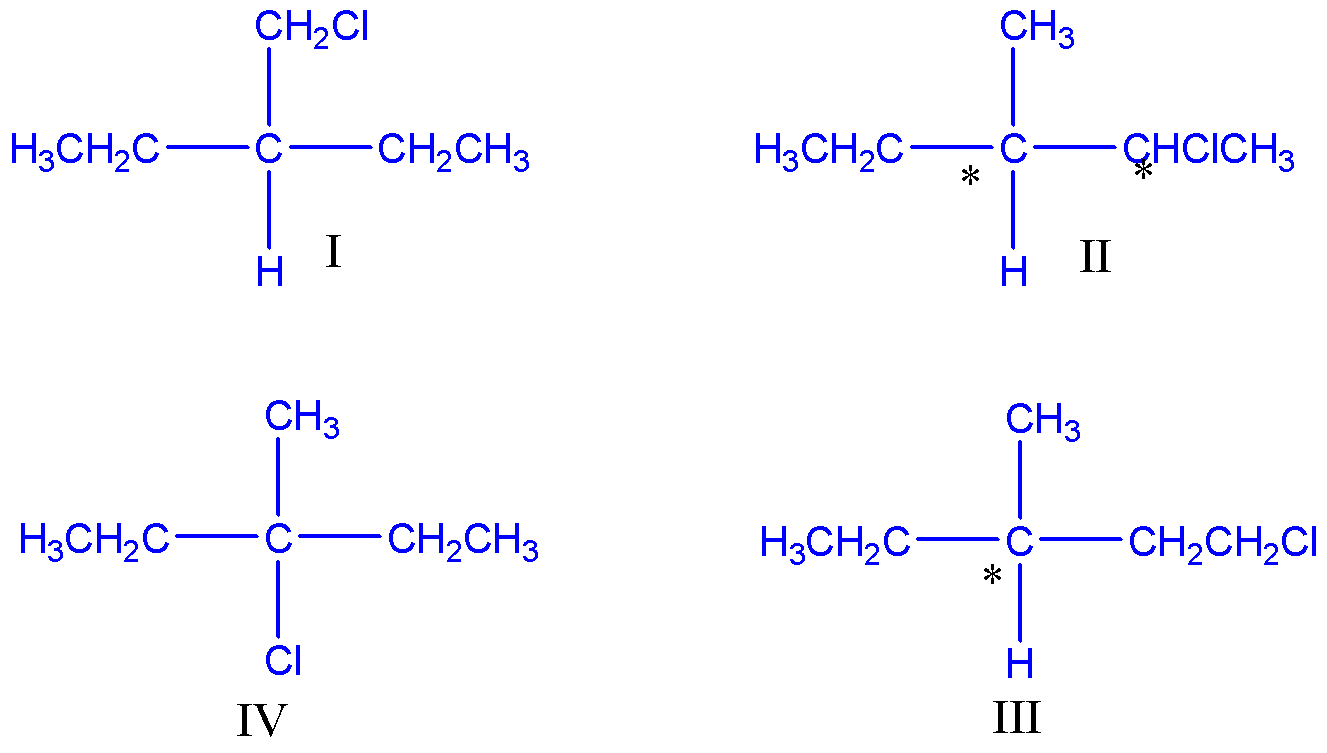Strucrure I and IV have no chiral centers, II has 2 chiral centres and III has one. No. of stereoisomers = 22= 4 and 21=2 for II and III respectively. That’s why the total no. of possible isomers will be 1+4+2+1= 8.In the Newman projection of 2,2-dimethylbutane X and Y respectively can be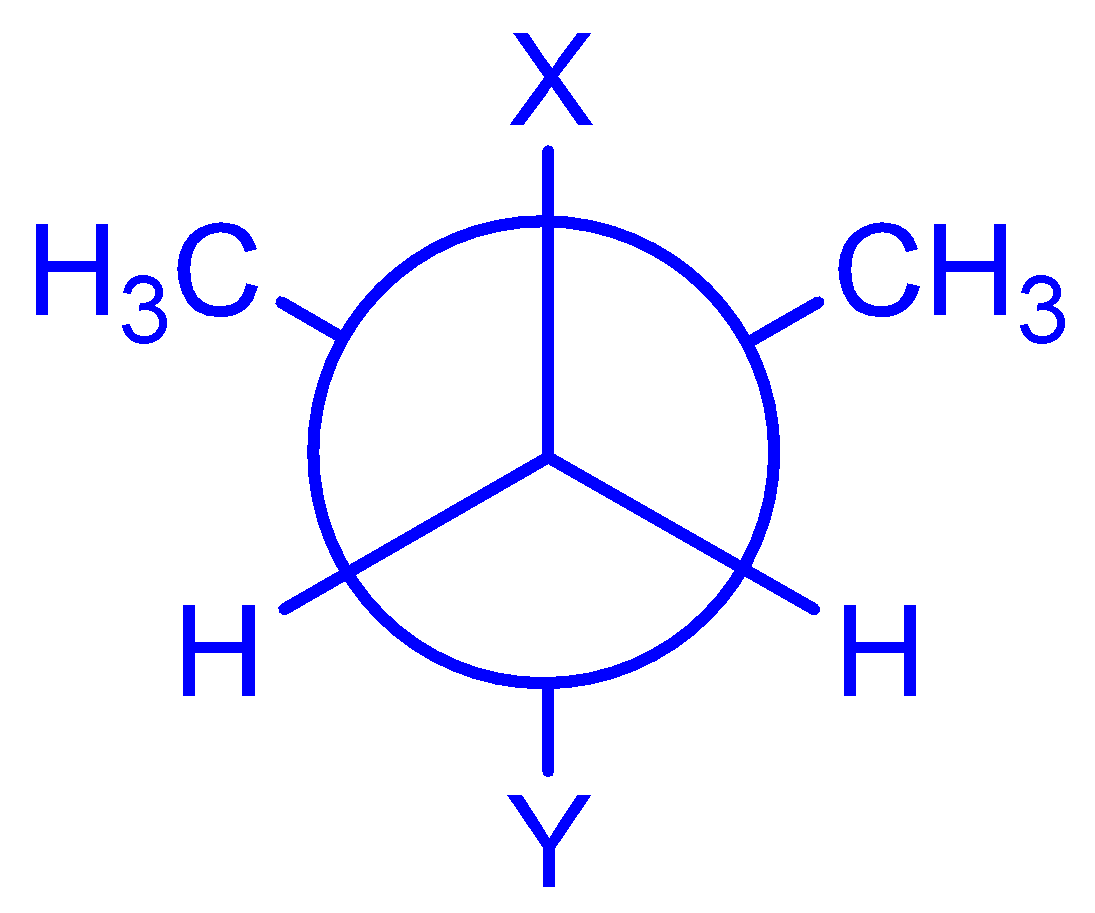H and HH and C2H5C2H5  and HCH3 and CH3Ans: d)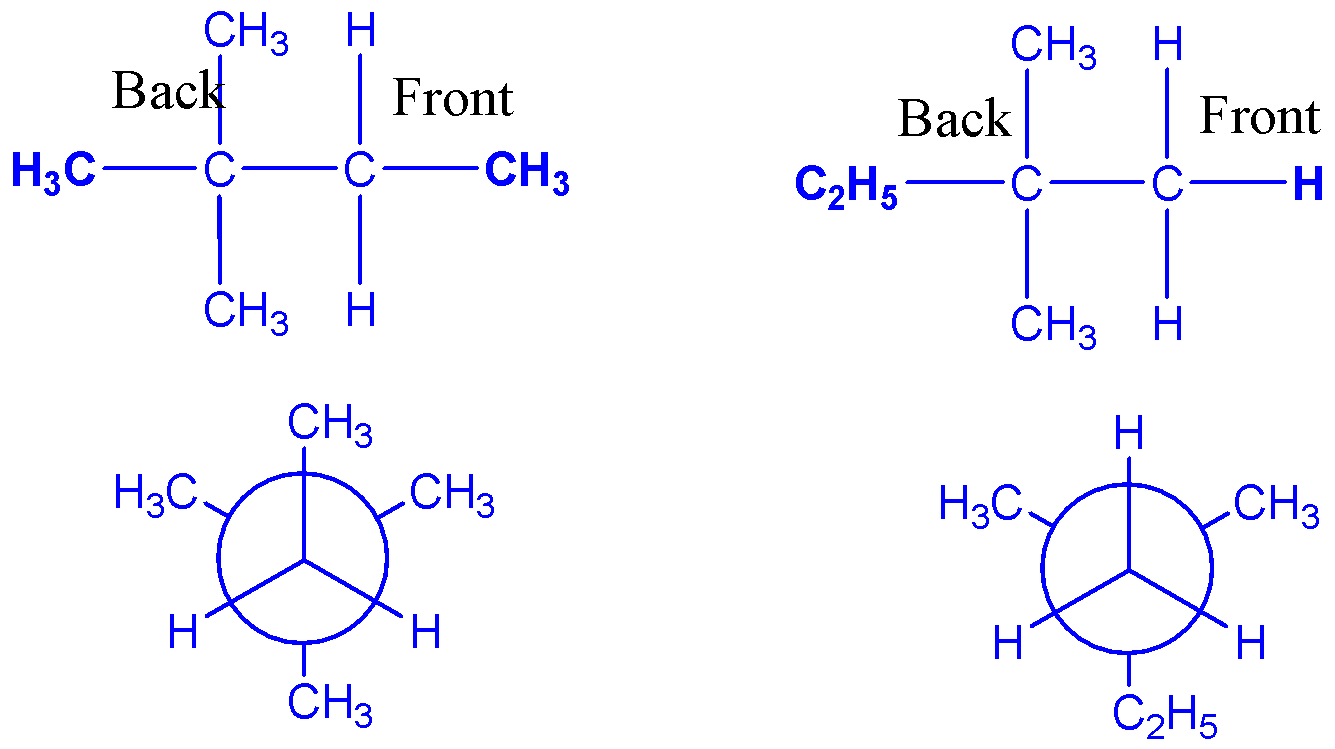The total no. of cyclic isomers possible for a hydrocarbon with the molecular formula C4H6 is ____ Ans: Double bond equivalent = 2 x 4 – 6 = 2So, there will either be 1 double bond + 1 ring or 2 rings which is possible in the following 4 ways: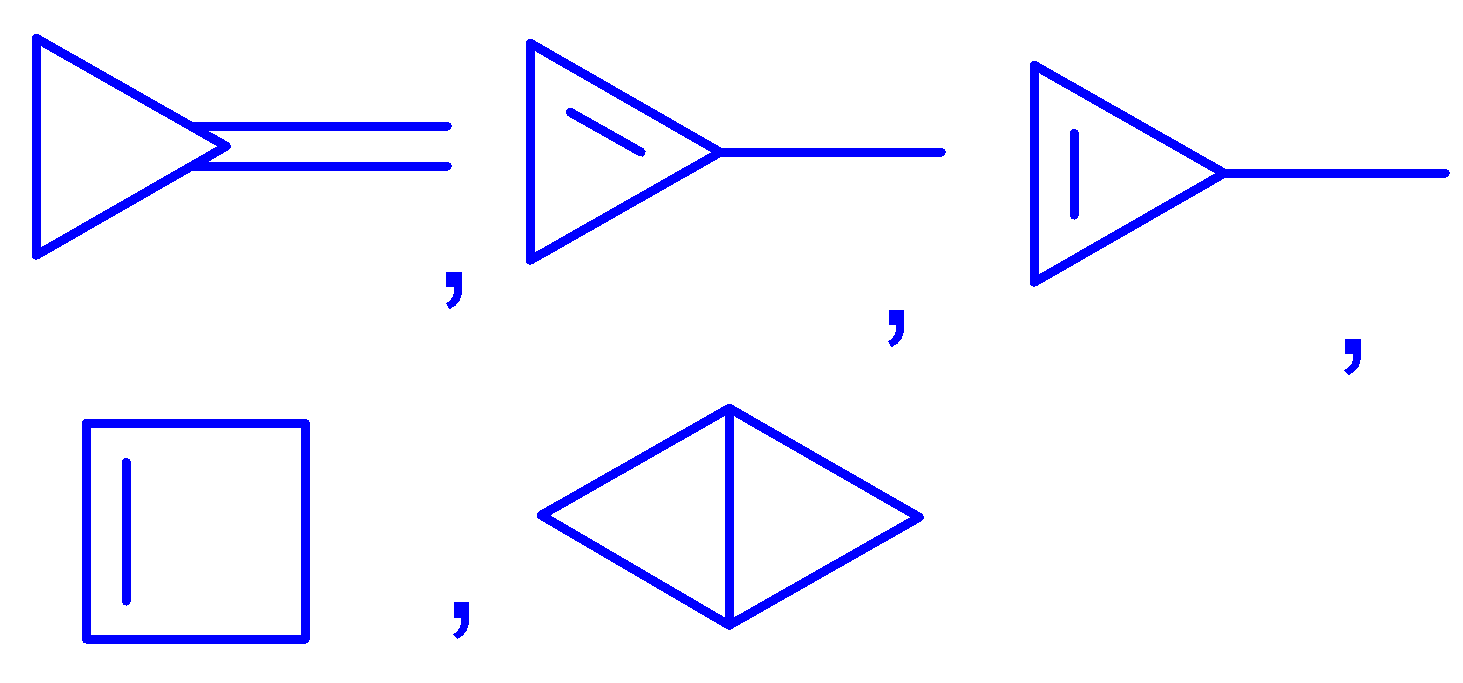The IUPAC name of the following compound is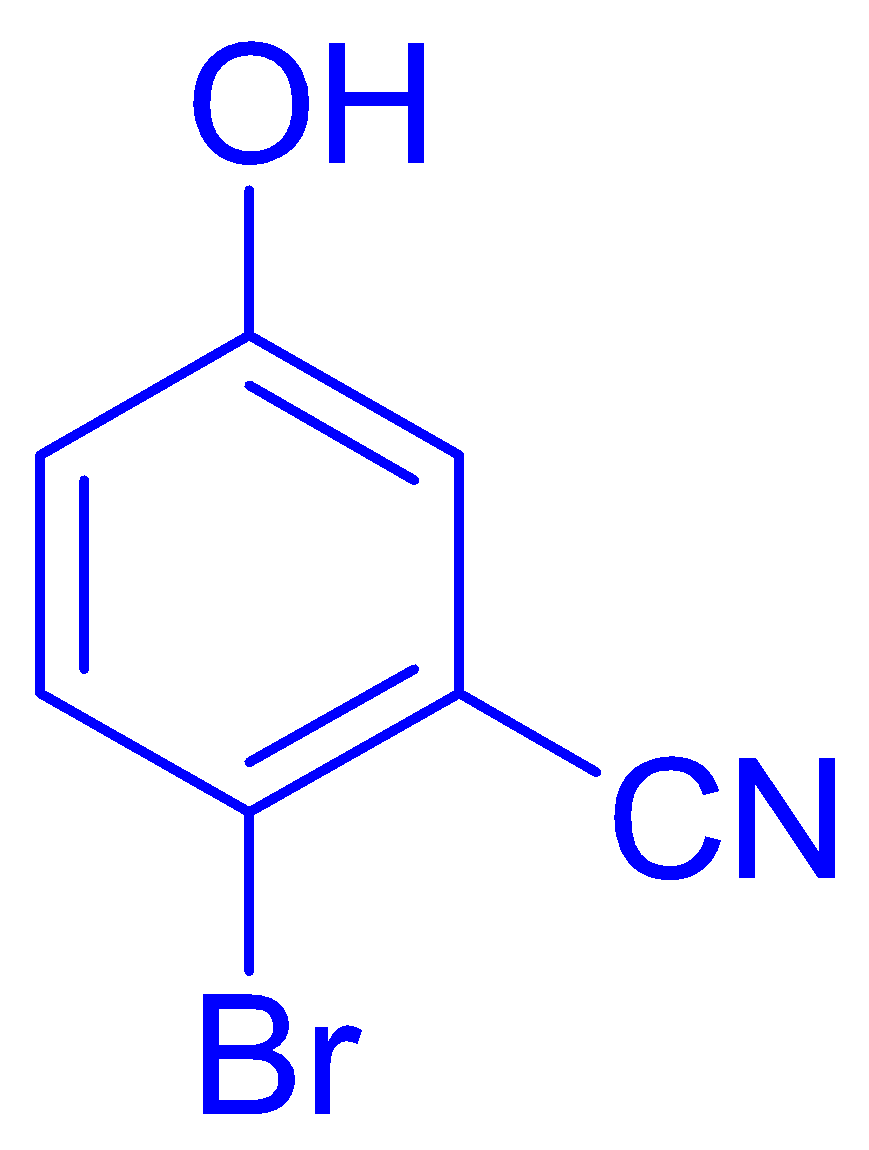4-Bromo-3-cyanophenol2-Bromo-5-hydroxybenzonitrile2-Cyano-4-hydroxybromobenzene6-bromo-3-hydroxybenzonitrileAns: (b) -CN is the principal functional group. If we start numbering anti-clockwise then, OH will get position 3 and Br will get 5 and if we do it clockwise then Br will get 2 and OH will get 5. So, the lowest set of positions (2,5) < (3,5) is possible only if we do the numbering clockwise.6.)   The possible no. of cyclic structural as well as stereo isomers for the mol. Formula C5H10    is ____Ans: D.B.E. = (5 x 2 – 10)/2 + 1 = 1. So, only one ring is possible.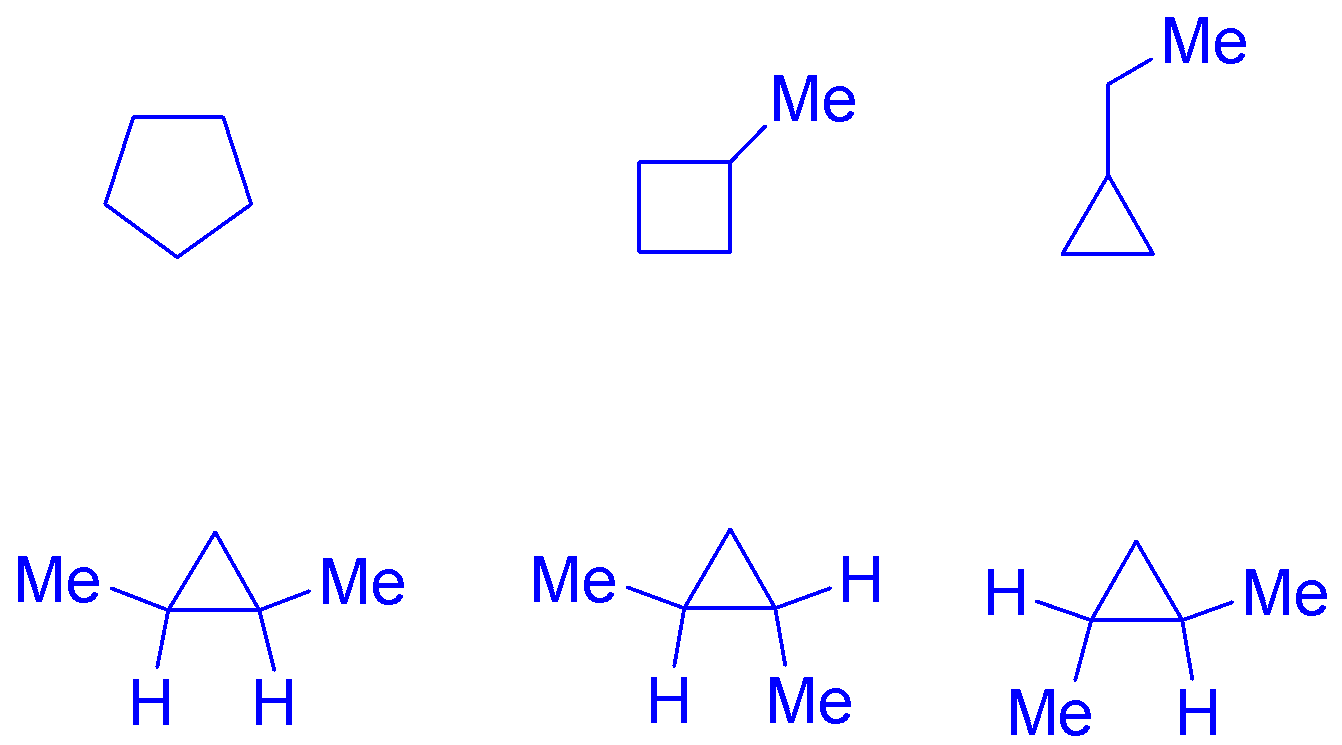The correct statement(s) about the compound given below is (are)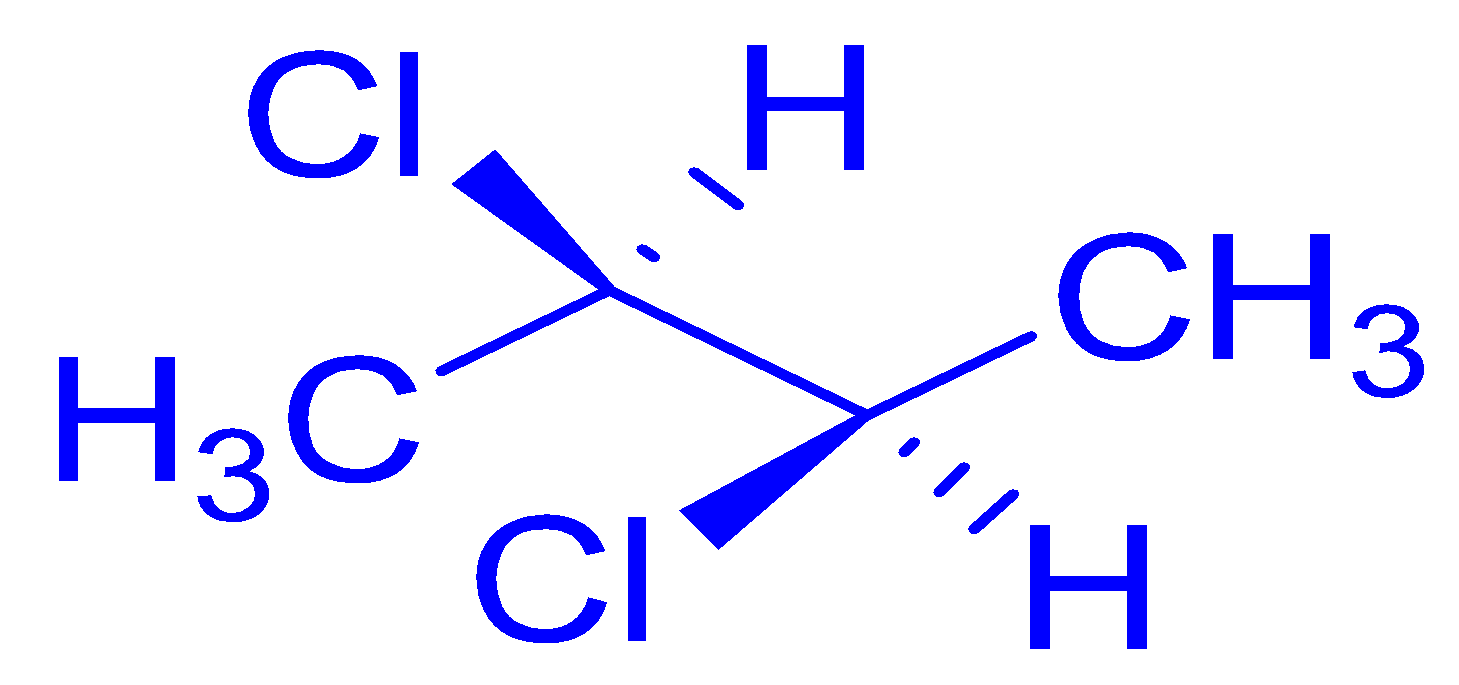The compound is optically active.The compound possesses centre of symmetry.The compound possesses plane of symmetry.The compound possesses of symmetry.Ans:  a)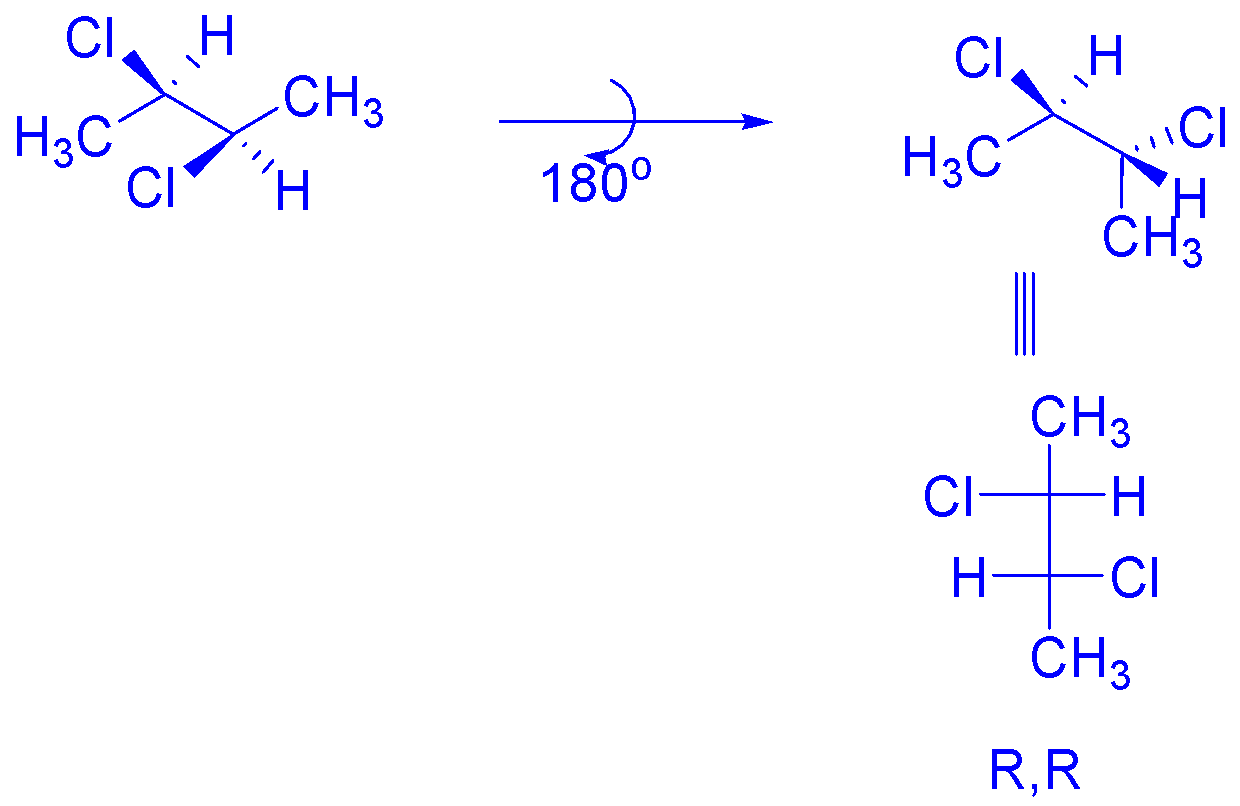The correct statement concerning E, F and G are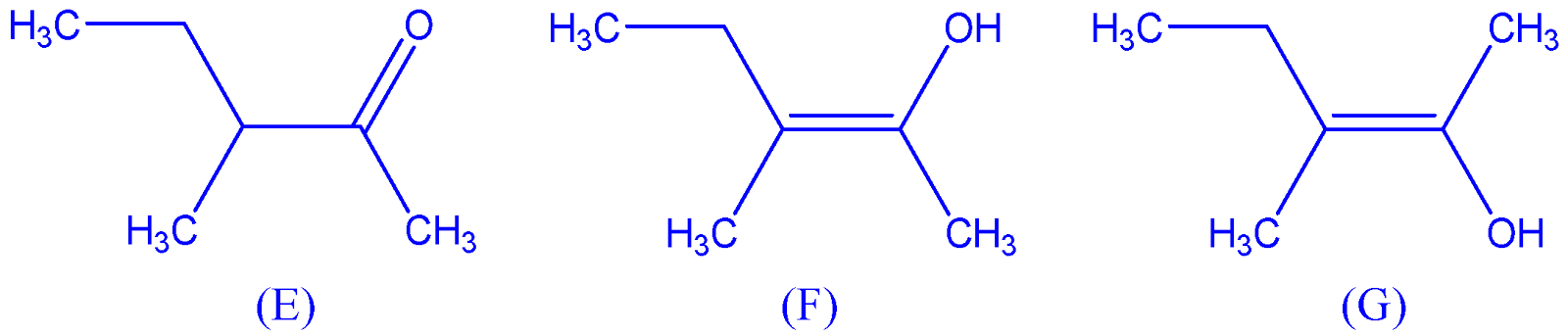E, F and G are resonance structures.E, F and E, G are tautomers.F and G are geometrical isomers.F and G are diastereomers.Ans: b), c) and d)